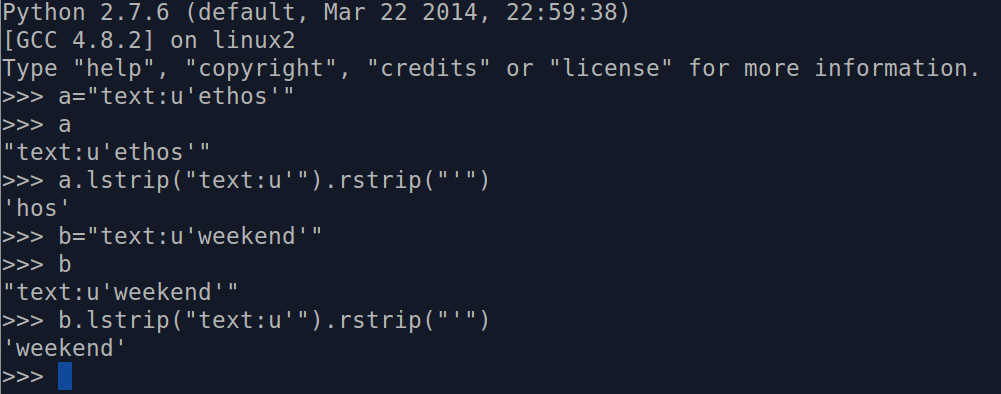# 前言

## 音频手机快速刷单词

### 改进

Github:再要你命3000手机刷词版-revised－20141205.pdf

# 技术实现细节

#### Excel生成TeX文件


# -*- coding: utf-8 -*-
"""
Created on Tue Oct 21 23:07:19 2014

@author: famer
"""

# this is the script to create a pdf file that can be read on smartphone
# original date is is in an excel called 3k.xls
# to create the pdf we use Latex

import xlrd

data = xlrd.open_workbook('3k.xls')

def createtex(i):
table = data.sheet_by_index(i)

texname=str(table.cell(0,0)).lstrip("text:u'").rstrip("'")

file= open (texname+".tex","wr")

file.write("%!TEX program=xelatex \n")
file.write("%!TEX root=3k.tex \n")
file.write("\chapter{ %s} \n" %texname )
file.write("\\begin{enumerate} \n")

nrows = table.nrows

for i in range(1,nrows):
word_eng=str(table.cell(i,0)).lstrip("text:u'").rstrip("'")
file.write("\item %s \\\\  \n" % word_eng)
word_chn=str(table.cell(i,1)).decode("unicode_escape").encode("utf8").lstrip("text:u'").rstrip("'")
file.write("%s \n" % word_chn)

file.write("\end{enumerate} \n")

file.close()

if __name__=="__main__":
for i in range(29,33):
createtex(i)
print "Done"



####　LaTeX文件生成

##补记


word_eng=str(table.cell(i,0)).lstrip("text:u'").rstrip("'")

word_chn=str(table.cell(i,1)).decode("unicode_escape").encode("utf8").lstrip("text:u'").rstrip("'")




# -*- coding: utf-8 -*-
"""
Created on Tue Oct 21 23:07:19 2014

@author: famer
"""

# this is the script to create a pdf file that can be read on smartphoe
# original date is is in an excel called 3k.xls
# to create the pdf we use Latex

import xlrd

data = xlrd.open_workbook('3k.xls')

def createtex(i):
table = data.sheet_by_index(i)

texname=str(table.cell(0,0)).lstrip("text:u'").rstrip("'")

file= open (texname+".tex","wr")

file.write("%!TEX program=xelatex \n")
file.write("%!TEX root=3k.tex \n")
file.write("\chapter{ %s} \n" %texname )
file.write("\\begin{enumerate} \n")

nrows = table.nrows

for i in range(1,nrows):
word_eng=table.cell_value(i,0)
file.write("\item %s \\\\  \n" % word_eng)

word_chn=table.cell_value(i,1)
file.write("%s \n" % word_chn)

file.write("\end{enumerate} \n")

file.close()

if __name__=="__main__":
for i in range(29,33):
createtex(i)
print "Done"




word_eng=table.cell_value(i,0)

word_chn=table.cell_value(i,1)



Python不愧是自带电池的语言，使用内置方法而不是自己生搬硬套一个。

text:u’ethos’Python 2.7.6 (default, Mar 22 2014, 22:59:38)
[GCC 4.8.2] on linux2
>>> a="text:u'ethos'"
>>> a
"text:u'ethos'"
>>> a.lstrip("text:u'").rstrip("'")
'hos'
>>> b="text:u'weekend'"
>>> b
"text:u'weekend'"
>>> b.lstrip("text:u'").rstrip("'")
'weekend'




>>> '   spacious   '.lstrip()
'spacious   '
>>> 'www.example.com'.lstrip('cmowz.')
'example.com'



"text:u'weekend'"[7:-1]



Written on October 21, 2014